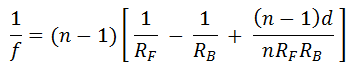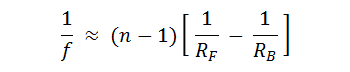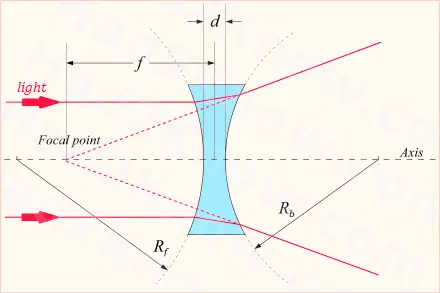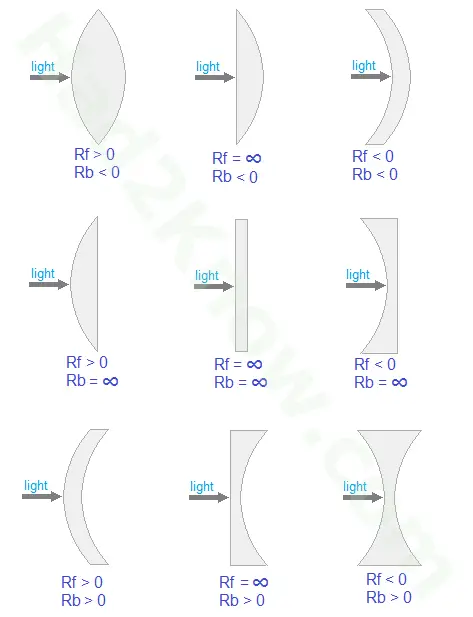# Focal Length Calculator

 Focal Distance Calculator(Enter "inf" for infinity.) RF RB Lens Thickness, d Refractive Index, n Focal Legth, f =

In optics, the focal power of a lens is the reciprocal of the focal length. For a lens whose curves are circular arcs, the focal distance in air depends on the radius of curvature of the front of the lens (the side nearest the light source), the radius of curvature of the back side of the lens (farthest from the light source), the thickness of the lens (measured at the center), and the refractive index of the lens material. For a lens in air, the equation iswhere f is the focal distance, RF is the front radius, RB is the back radius, d is the lens thickness, and n is the refractive index.

For a thin lens, the equation is often simplified to### Focal Distance DiagramThe diagram above shows the location of the focal point of the lens for a biconcave lens. If the focal point is on the same side as the light source, the focal distance is negative and the lens is divergent. If the focal point is behind the lens the focal length is positive and the lens is convergent. If the focal length is infinite, the lens has no power and is neither divergent nor convergent.

### Types of Lenses and Their RF and RB ValuesWhen plugging the values of RF and RB into the focal length equation, sign matters! If the center of curvature is away from light source, the radius is positive. If the center of curvature is near light source, the radius is negative. If either side is flat, the radius is infinite and the value of 1/R is 0.

### Refractive Indices

The refractive index of a medium is a dimensionless number that indicates the focusing power of a lens made from that medium. For most transparent materials, the index of refraction is greater than 1 and less than 2, though some materials have an index of 2 or more. The refractive index of a total vacuum is exactly 1 by definition. Here is a list of the refraction indices of common liquids and solids

Liquids
Water at 20°C: 1.333
Ethanol at 20°C: 1.361
50% Sugar Solution in Water: 1.420
Benzene at 20°C: 1.501
Silicone Oil at 20°C: 1.520

Solids
Ice at -20°C: 1.31
Pyrex: 1.47
Acrylic Glass: 1.49
Pure Crown Glass 1.50 - 1.54
Pure Flint Glass: 1.60 - 1.62

### Example

The radius of curvature of a lens is 130 cm at the front and -250 cm at the back. The thickness of the lens is 0.4 cm and the refractive index of the material is 1.37. The values of the key variables are

RF = 130
RB = -250
d = 0.4
n = 1.37

The inverse of the focal length is given by the equation

1/f = (0.37)[1/130 + 1/250 - 0.37*0.4/(1.37*130*250)]
= 0.004324924

And so the focal length is

f = 1/0.004324924 = 231.218 cm.